Next: 3. The Silicon/Silicon-Dioxide Interface Up: 2. Simulation of Semiconductor Previous: 2.3 Carrier Generation and

Subsections

# 2.4 Quantum Mechanical Effects

The classical semiconductor device equations from Section 2.1 imply that the mobile carriers, electrons and holes, behave like classical particles in the semiconductor. For large device dimensions this assumption gives very good results, but for small device geometries quantum mechanical effects like the quantum mechanical tunneling, described in Section 5.3, and the quantum mechanical confinement gain importance. The latter effect leads to a reduction of allowed states for electrons and holes near a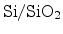interface. In classical device simulations using the drift-diffusion approximation the peak of the electron concentration in the channel of a turned on n-channel MOSFET is calculated to be directly at theinterface. This is not correct as the number of allowed states is drastically reduced close to the interface and therefore the peak of the carrier concentration lies several angstroms away from the interface .

## 2.4.1 Quantum Confinement

For the modeling of NBTI the carrier concentration close to theinterface plays an important role (Section 6.4.4). The use of quantum confinement models reduces this carrier concentration and might have significant influence on the NBTI model used.

In classical device simulators quantum confinement is often accounted for by using additional quantum correction models. These models locally change the carrier density-of-states [10,11] or they modify the conduction band edge close to the interface .

### 2.4.1.1 Density of States Correction

In classical device simulation the density-of-states (DOS) in homogeneous materials is modeled as a constant value throughout the device. In order to describe the quantum mechanical confinement a distance-dependent reduction of the DOS at theinterface has been proposed in [10,11]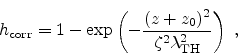(2.106)

where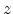is the distance to theinterface andshifts the whole function relative to the interface.is a newly introduced parameter which enables the variation of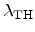for calibration purposes. The symboldenotes the thermal wavelength which is given by(2.107)

where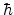is the reduced Planck constant,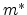is the effective carrier mass,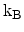the Boltzmann constant, and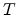the temperature. The resulting DOS,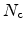, is then calculated from the classical DOS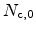, which is normally modeled as a constant throughout the semiconductor, with the correction factor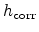as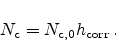(2.108)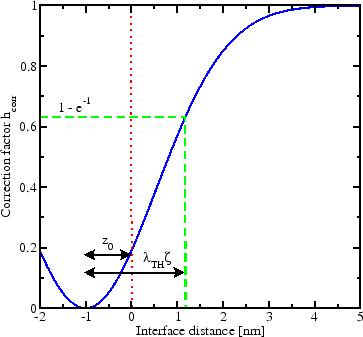The interplay of the different parameters and the distance to theinterface is schematically depicted in Figure 2.5. The parameteris important, because with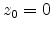the DOS at the interface becomes zero. This would cause numerical problems and reduce the convergence of the numerical solver. A positive number ofshifts the correction function towards the dielectric, approximately considering wave function penetration. The value of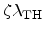defines the effective depth of the correction. A high value, which can be achieved with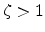, leads to a reduction of the DOS even deep in the substrate.

Note that the correction factor does not depend on the bias and the band edge energies are not influenced. Hence, the model can be evaluated in a preprocessing step and does not impose any additional computational burden during iteration steps.

### 2.4.1.2 Conduction Band Edge CorrectionAn alternative approach is based on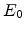, the first eigenvalue of the triangular energy well, as seen in Figure 2.6. This model was proposed by van Dort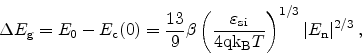(2.109)

where the proportionality factor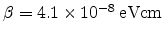is is found from the observed threshold voltage shift at high doping levels ,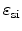is the permittivity of silicon, and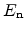is the electric field at theinterface perpendicular to the interface.

The value of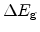is multiplied with a distance-dependent weight function which has been introduced by Selberherr  for the modeling of surface roughness scattering in MOSFETs. The function is of the following form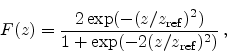(2.110)

where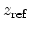is the scaling factor for the interface distance. Thus, the resulting band edge energy with van Dort's quantum correction of the classical band edge energy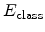reads as follows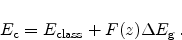(2.111)

Figure 2.6 depicts the distance dependent weight function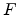and the band edge energy for both, the classical approach and after quantum correction with van Dort's method.

### 2.4.1.3 Evaluation of Quantum Correction Models

For the evaluation of the quantum correction models a state-of-the-art three-dimensional n-channel FinFET device structure was chosen. The device geometry can be seen in Figure 2.7.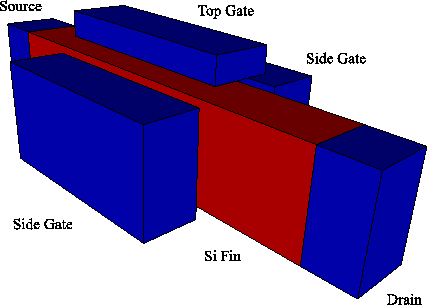The silicon fin has a cross section area of 6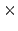10nm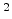. The gate length is 20nm with a gate oxide thickness of 1.5nm. The source and drain regions are heavily n-type doped whereas the channel itself remains undoped.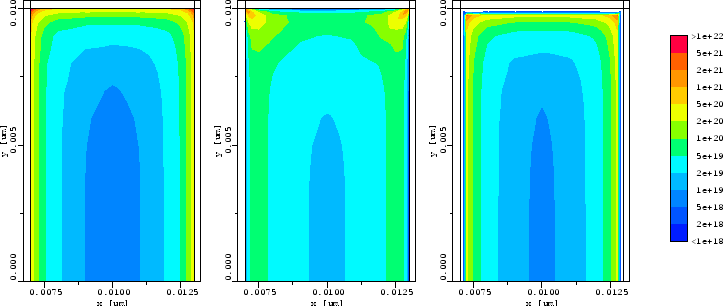Figure 2.8 depicts the electron concentration in a two-dimensional cut through the silicon fin in the middle of the channels. The gates are biased at 0.9V with the source and drain contacts grounded. The classical simulation using the drift-diffusion approximation gives the highest magnitude of the electron concentration at theinterfaces below the gate contacts. It can be seen that the peak electron concentration is found in the top corners, as two respective gates couple to the channel, each of them attracting carriers. With the quantum confinement correction models the maximum carrier concentration is moved to the inside of the fin by a distance depending on the chosen model and its calibration parameters.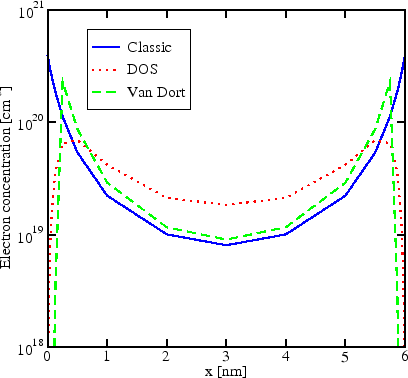Figure 2.9 depicts a one-dimensional cut through the fin displaying the carrier concentrations for the different models for the same bias conditions. Comparing with Figure 2.10 reveals that qualitatively the DOS correction model delivers better results.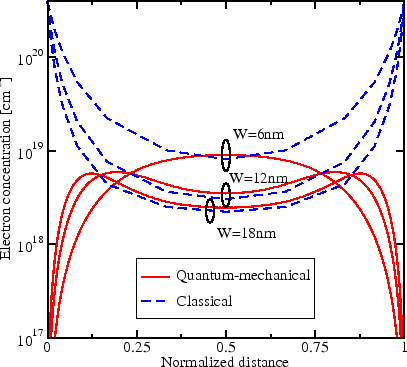A comparison of FinFETs with different fin widths can be seen in Figure 2.10. It shows the electron concentration across the fin simulated with both, the classical drift-diffusion model and a Schrödinger solver, respectively. The fin widths are 6, 12, and 18nm. At a fin width of 6nm the electron concentration has its maximum in the center of the fin. This shape of the carrier concentration cannot be reproduced by the quantum correction models. With larger widths the maximum moves to the interfaces enabling a better fit of the correction models.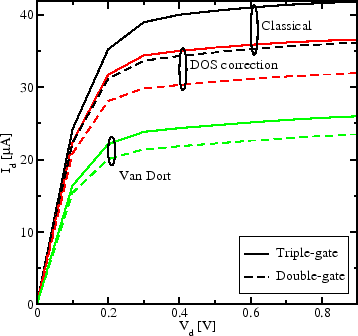The channels in the silicon fin are displaced from the surface to the inside of the silicon and thus the drive current is reduced. Figure 2.11 depicts the drain current for a gate voltage of 0.9V and different quantum correction mechanisms. Additionally to the triple-gate device the simulation has been performed with a double-gate structure, where the top gate from Figure 2.7 has been replaced with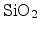. Simulation of the double-gate structure shows a reduced output current by a factor of approximately 20% due to the formation of only two channels.

Quantum correction leads to a considerable reduction of the saturation current. The DOS correction model yields reasonable results, but since it does not account for the band bending it must be calibrated for each bias point. Van Dort's model completely fails to reproduce the carrier concentration in the channel which may be due to the assumption of a triangular energy well. This assumption might be a too crude estimation for extremely thin channels. Therefore, these models can be very well used to describe the current reduction in very thin channel devices, but not when the shape of the carrier concentration is important. Here, the solution of Schrödinger's equation is necessary for accurate simulation of the carrier concentration.Next: 3. The Silicon/Silicon-Dioxide Interface Up: 2. Simulation of Semiconductor Previous: 2.3 Carrier Generation and

R. Entner: Modeling and Simulation of Negative Bias Temperature Instability